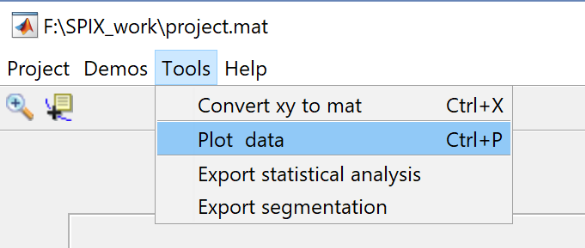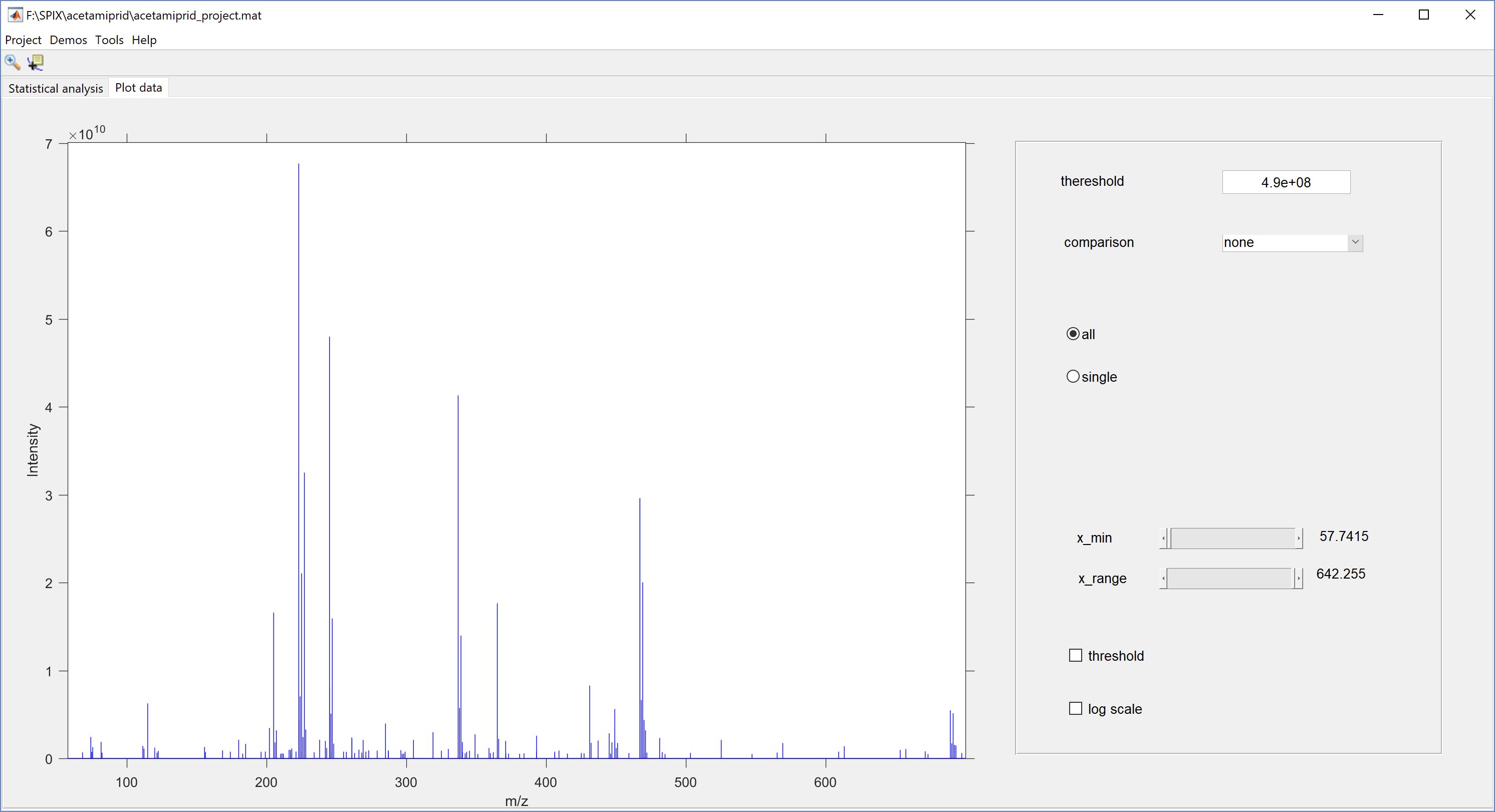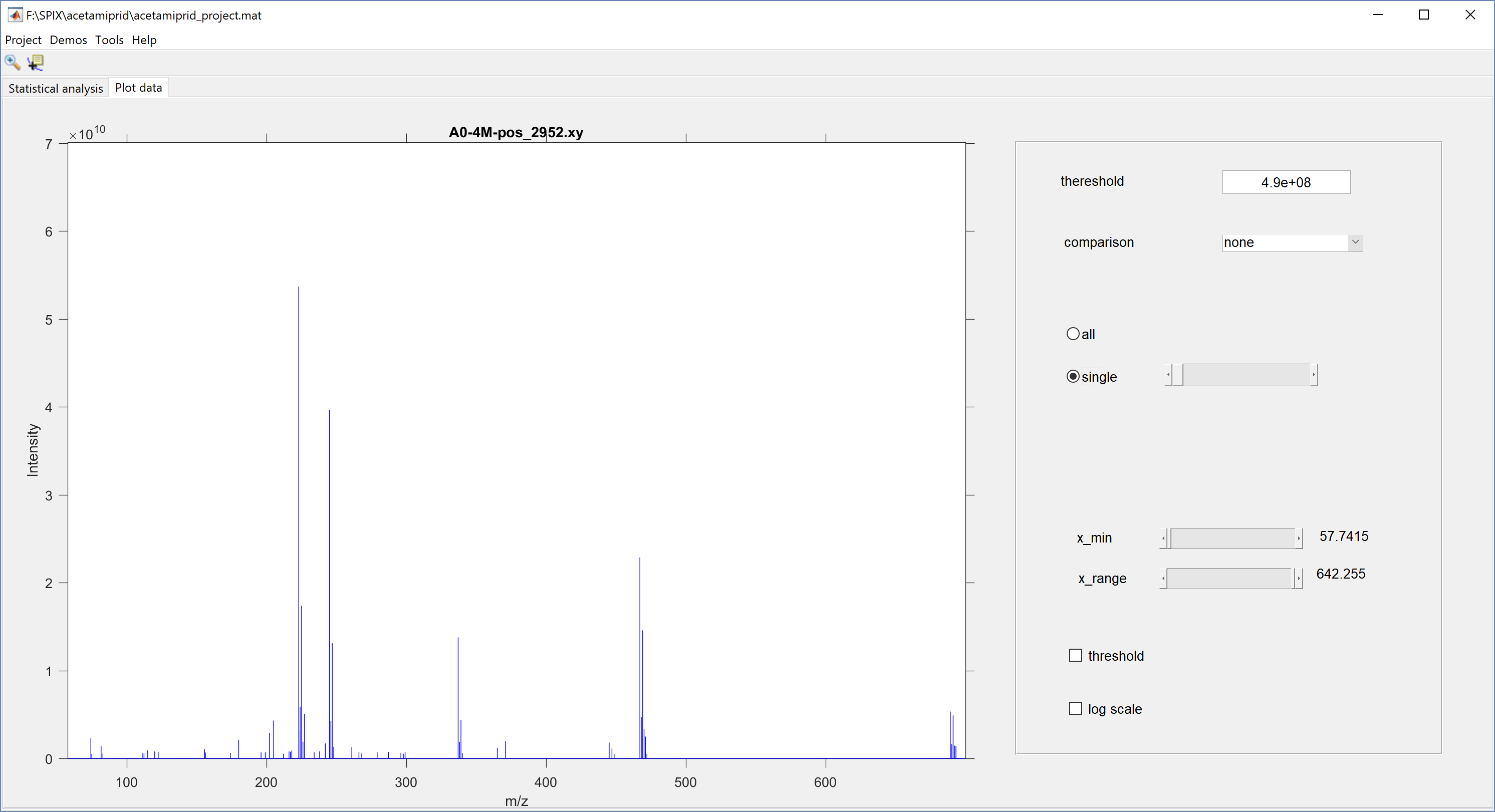Select Tools/Plot data in the main menuBy default, the complete raw data, i.e. all spectra, are displayed on the same plot:Select single to plot each spectrum separately,Use the slider in order to change the range of $$m/z$$ values. For instance, set $$x_{\rm min}=200$$ and $$x_{\rm range}=300$$ if you want to display spectra between $$m/z=200$$ and $$m/z=500$$.